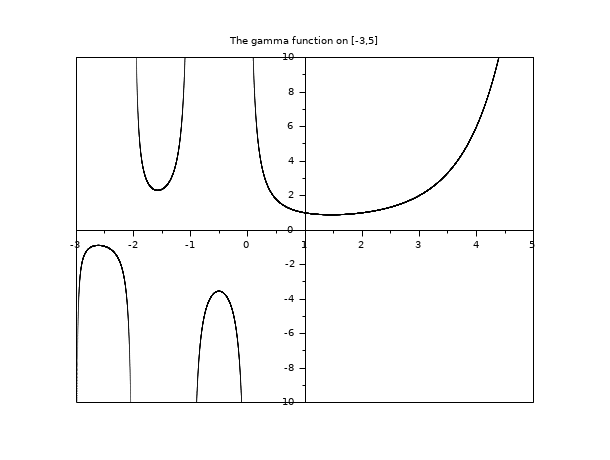Scilab Home page | Wiki | Bug tracker | Forge | Mailing list archives | ATOMS | File exchange
Scilab 6.0.2
Change language to: Français - Português - 日本語 - Русский
Scilab Help >> Special Functions > gamma

gamma

The gamma function.

y = gamma(x)

Arguments

x

scalar, vector, matrix, or hypermatrix of real numbers.gamma can be overloaded for complex numbers or of lists, tlists or mlists.
y

real vector or matrix with same size than x.

Description

gamma(x) evaluates the gamma function at all the elements of x. The gamma function is defined by :and generalizes the factorial function for real numbers (gamma(u+1) = u*gamma(u)).

Examples

// simple examples
gamma(0.5)
gamma(6)-prod(1:5)
// the graph of the Gamma function on [a,b]
a = -3; b = 5;
x = linspace(a,b,40000);
y = gamma(x);
clf()
plot2d(x, y, style=0, axesflag=5, rect=[a, -10, b, 10])
xtitle("The gamma function on ["+string(a)+","+string(b)+"]")
show_window()• gammaln — The logarithm of gamma function.
• dlgamma — derivative of gammaln function, psi function
• factorial — The factorial function

History

 Version Description 5.4.0 Overloading allowed for list, mlist, tlist and hypermatrix types. 6.0.2 The input can now be an hypermatrix. gamma can now be overloaded for complex numbers.